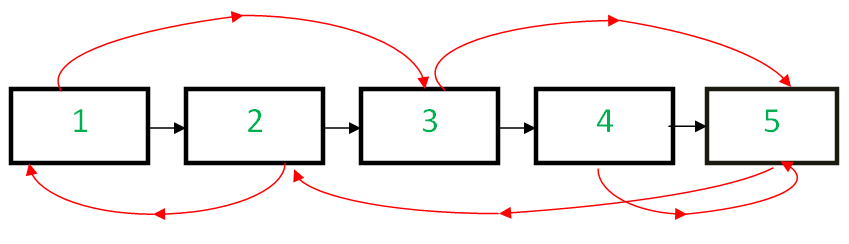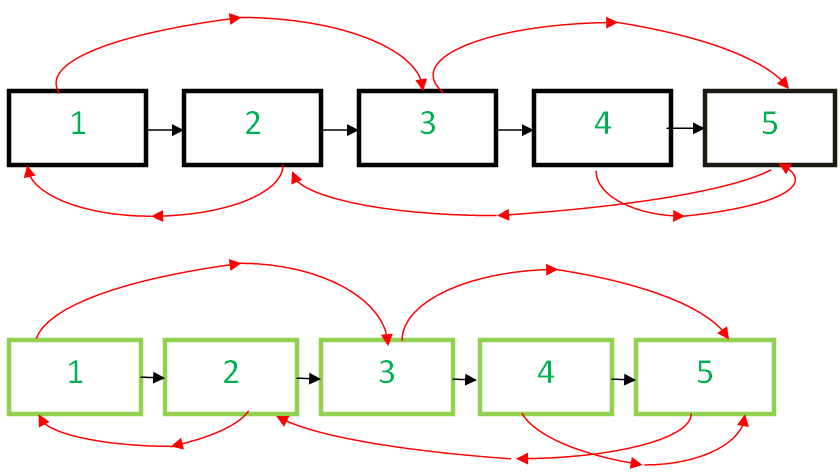# Java Program For Cloning A Linked List With Next And Random Pointer In O(1) Space

Given a linked list having two pointers in each node. The first one points to the next node of the list, however, the other pointer is random and can point to any node of the list. Write a program that clones the given list in O(1) space, i.e., without any extra space.
Examples:

`Input : Head of the below-linked list````Output :
A new linked list identical to the original list.```

In the previous posts Set-1 and Set-2 various methods are discussed, and O(n) space complexity implementation is also available.
In this post, we’ll be implementing an algorithm that’d require no additional space as discussed in Set-1.
Below is the Algorithm:

• Create the copy of node 1 and insert it between node 1 & node 2 in the original Linked List, create a copy of 2 and insert it between 2 & 3. Continue in this fashion, add the copy of N after the Nth node

• Now copy the random link in this fashion

``` original->next->random= original->random->next;  /*TRAVERSE
TWO NODES*/```
• This works because original->next is nothing but a copy of the original and Original->random->next is nothing but a copy of the random.

• Now restore the original and copy linked lists in this fashion in a single loop.

```original->next = original->next->next;
copy->next = copy->next->next;```
• Ensure that original->next is NULL and return the cloned listBelow is the implementation.

## Java

 `// Java program to clone a linked list with next` `// and arbit pointers in O(n) time` `class` `GfG {`   `    ``// Structure of linked list Node` `    ``static` `class` `Node {` `        ``int` `data;` `        ``Node next, random;` `        ``Node(``int` `x)` `        ``{` `            ``data = x;` `            ``next = random = ``null``;` `        ``}` `    ``}`   `    ``// Utility function to print the list.` `    ``static` `void` `print(Node start)` `    ``{` `        ``Node ptr = start;` `        ``while` `(ptr != ``null``) {` `            ``System.out.println(``"Data = "` `+ ptr.data` `                               ``+ ``", Random = "` `                               ``+ ptr.random.data);` `            ``ptr = ptr.next;` `        ``}` `    ``}`   `    ``// This function clones a given` `    ``// linked list in O(1) space` `    ``static` `Node clone(Node start)` `    ``{` `        ``Node curr = start, temp = ``null``;`   `        ``// insert additional node after` `        ``// every node of original list` `        ``while` `(curr != ``null``) {` `            ``temp = curr.next;`   `            ``// Inserting node` `            ``curr.next = ``new` `Node(curr.data);` `            ``curr.next.next = temp;` `            ``curr = temp;` `        ``}` `        ``curr = start;`   `        ``// adjust the random pointers of the` `        ``// newly added nodes` `        ``while` `(curr != ``null``) {` `            ``if` `(curr.next != ``null``)` `                ``curr.next.random = (curr.random != ``null``)` `                                       ``? curr.random.next` `                                       ``: curr.random;`   `            ``// move to the next newly added node by` `            ``// skipping an original node` `            ``curr = curr.next.next;                    ` `        ``}`   `        ``Node original = start, copy = start.next;`   `        ``// save the start of copied linked list` `        ``temp = copy;`   `        ``// now separate the original list and copied list` `        ``while` `(original != ``null``) {` `            ``original.next =original.next.next;`   `          ``copy.next = (copy.next != ``null``) ? copy.next.next` `                                            ``: copy.next;` `            ``original = original.next;` `            ``copy = copy.next;` `        ``}` `        ``return` `temp;` `    ``}`   `    ``// Driver code` `    ``public` `static` `void` `main(String[] args)` `    ``{` `        ``Node start = ``new` `Node(``1``);` `        ``start.next = ``new` `Node(``2``);` `        ``start.next.next = ``new` `Node(``3``);` `        ``start.next.next.next = ``new` `Node(``4``);` `        ``start.next.next.next.next = ``new` `Node(``5``);`   `        ``// 1's random points to 3` `        ``start.random = start.next.next;`   `        ``// 2's random points to 1` `        ``start.next.random = start;`   `        ``// 3's and 4's random points to 5` `        ``start.next.next.random = start.next.next.next.next;` `        ``start.next.next.next.random` `            ``= start.next.next.next.next;`   `        ``// 5's random points to 2` `        ``start.next.next.next.next.random = start.next;`   `        ``System.out.println(``"Original list : "``);` `        ``print(start);`   `        ``System.out.println(``"Cloned list : "``);` `        ``Node cloned_list = clone(start);` `        ``print(cloned_list);` `    ``}` `}`   `// This code is contributed by Prerna Saini.`

Output

```Original list :
Data = 1, Random  = 3
Data = 2, Random  = 1
Data = 3, Random  = 5
Data = 4, Random  = 5
Data = 5, Random  = 2

Cloned list :
Data = 1, Random  = 3
Data = 2, Random  = 1
Data = 3, Random  = 5
Data = 4, Random  = 5
Data = 5, Random  = 2```

Time Complexity: O(n), where n is the number of nodes in the given linked list.

Auxiliary Space: O(1), as no extra space is used. The n nodes which are inserted in between the nodes was already required to clone the list, so we can say that we did not use any extra space.

Please refer complete article on Clone a linked list with next and random pointer in O(1) space for more details!

Feeling lost in the world of random DSA topics, wasting time without progress? It's time for a change! Join our DSA course, where we'll guide you on an exciting journey to master DSA efficiently and on schedule.
Ready to dive in? Explore our Free Demo Content and join our DSA course, trusted by over 100,000 geeks!

Previous
Next